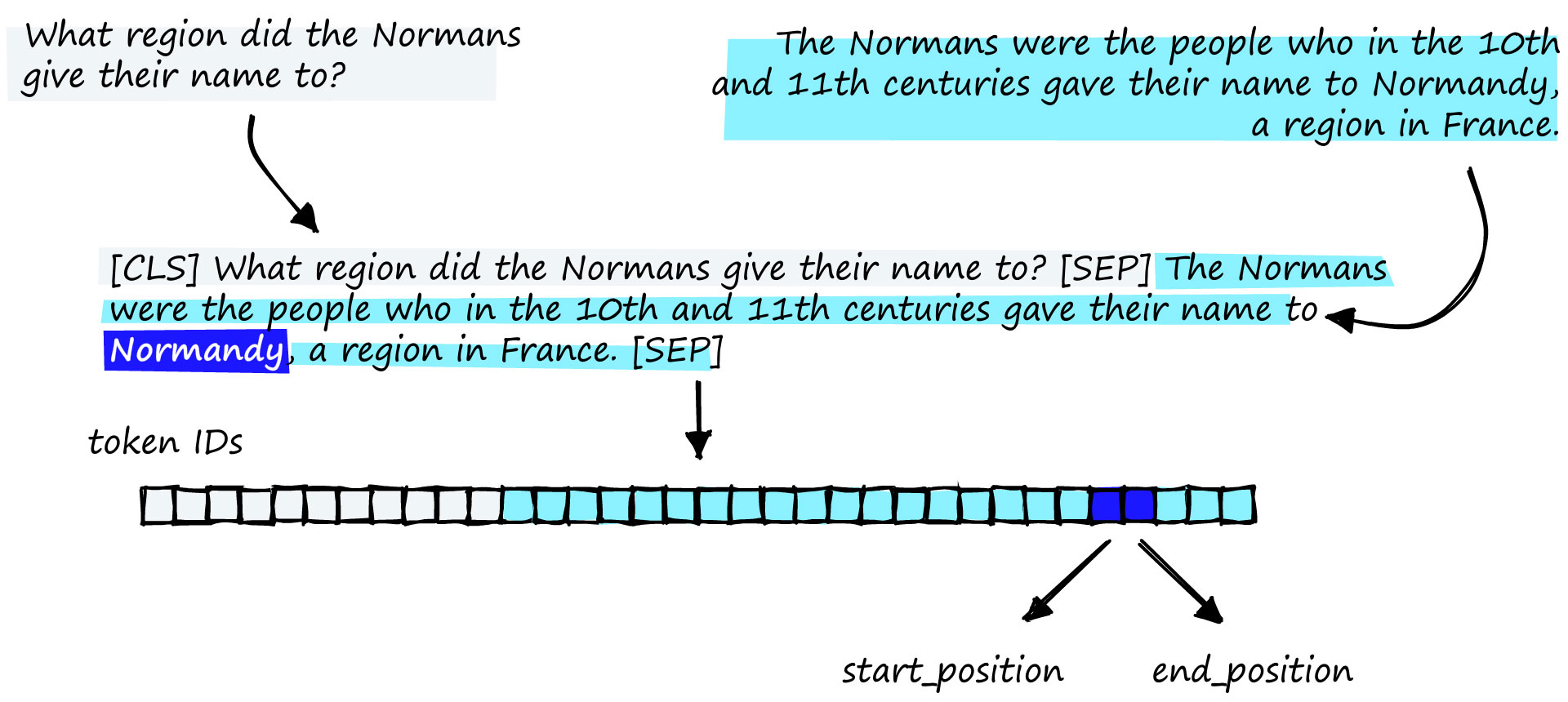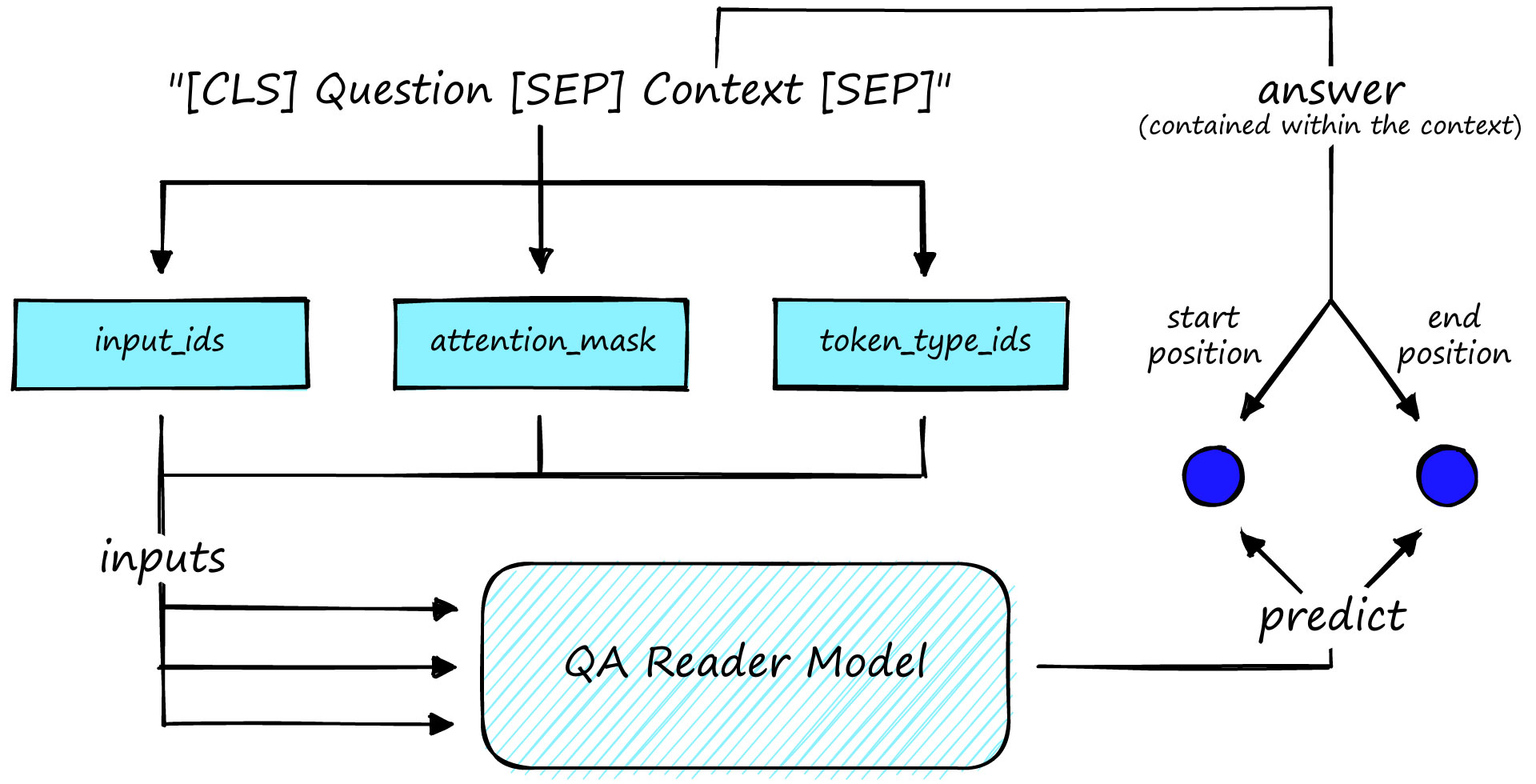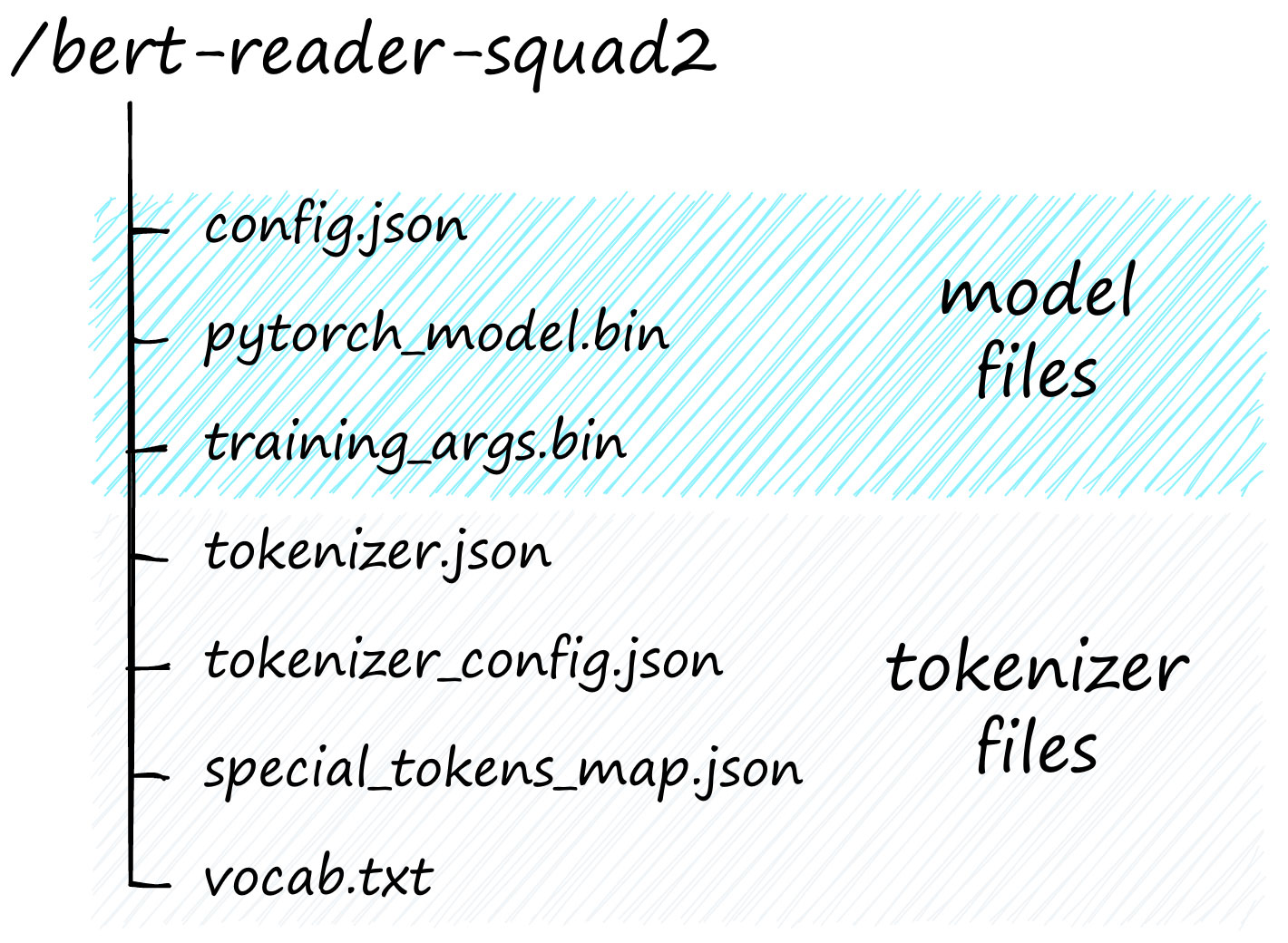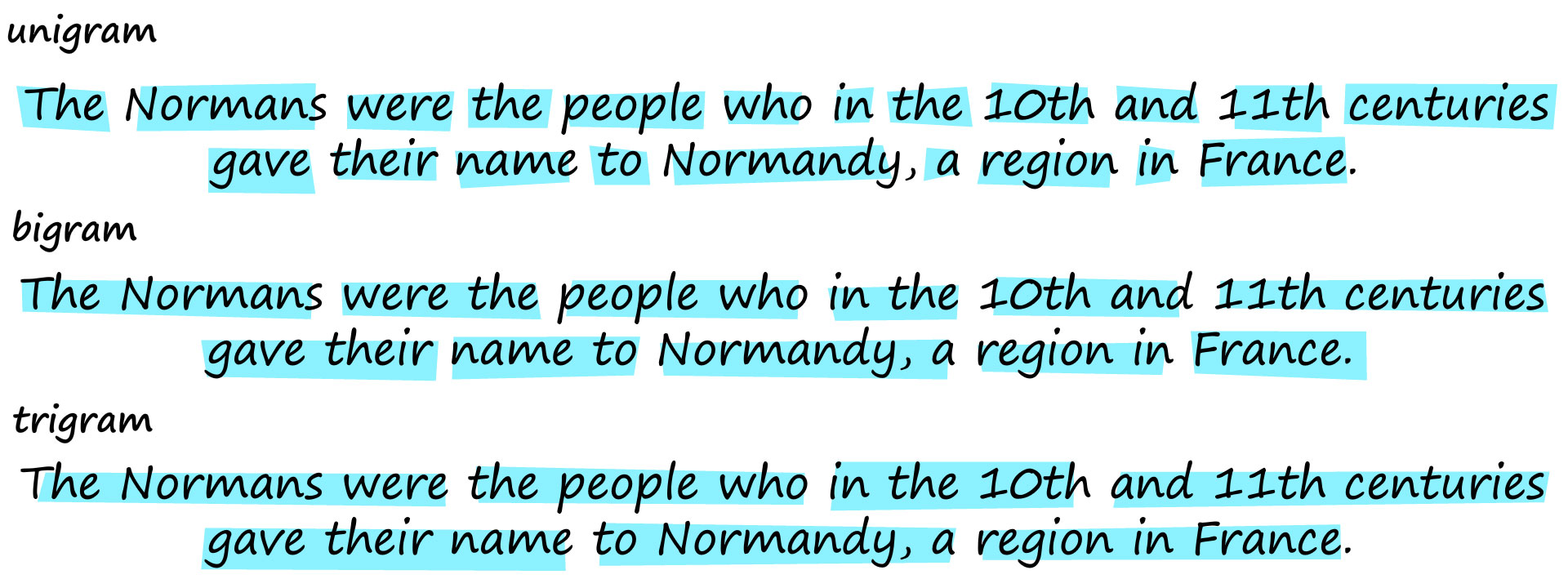# Reader Models for Open Domain Question-Answering

Open-domain question-answering (ODQA) is a wildly popular pipeline of databases and language models that allow us to ask a machine human-like questions and return comprehensible and even intelligent answers.

Despite the outward guise of simplicity, ODQA requires a reasonably advanced set of components placed together to enable the extractive Q&A functionality.

We call this extractive Q&A because the models are not generating an answer. Instead, the answer already exists but is hidden somewhere within potentially thousands, millions, or even more data sources.

By enabling extractive Q&A, we enable a more intelligent and efficient way to retrieve information from what can be massive stores of data.

ODQA relies on three components: the vector database, for storing encoded vector representations of the data we will search, a retriever to handle context and question encoding, and a reader model that consumes relevant retrieved contexts and identifies a shorter, more specific answer.

The reader is the final act in an ODQA pipeline; it takes the contexts returned by the vector database and retriever components and reads them. Our reader will then return what it believes to be the specific answer to our question.

To be exact, we don’t get the ‘specific answer’. The model is reading input IDs, which are integers representing words or subwords. So, rather than returning a human-readable text answer, it actually returns a span of input ID positions.Question (grey), context (cyan), and answer (blue). The model doesn’t read the strings. It reads token IDs, and so when outputting a prediction for the answer, it outputs a span of token IDs that it believes represent the answer.

To fine-tune a model, we need two inputs and two labels. The inputs are the question and a relevant context, and the labels are the answer’s start and end positions.Inputs (cyan) and target labels (answer start and end positions, blue). The start and end positions are the token positions from the encoded question-context input_ids tensor that represent the start and end of the answer (extracted from the context).

There isn’t much more to fine-tuning a reader model. It’s a relatively straightforward process. The most complex part is pre-processing the training data.

With our overview complete, let’s dive into the details and work through an actual training example.

## Implementation

There are more steps when training a reader model than just train the model. As mentioned, these other steps can prove to be the tricky part. In our case, we have three distinct steps.

2. Fine-tune the model
3. Evaluation

Without any further ado, let’s begin with the data.

We will be using the Stanford Question and Answering Dataset (SQuAD) for fine-tuning. We can download it with HuggingFace Datasets.

Looking at this, we have five features, of which we only care about question, context for the inputs, and answers for the labels.

We must make a few transformations to format the answers into the start and end token ID positions we need. We have answer_start, but this gives us the position within the context string that the answer begins. These positions are not what the model needs. Instead, it requires the start position using token ID indexes.

That is our main hurdle. To push through it, we will take three steps:

1. Tokenize the context.
2. Convert answer_start to a token ID index.
3. Find the end token index using the starting position and answer text.

Starting with tokenize the context, we first initialize a tokenizer using the HuggingFace Transformers library.

Then we tokenize our question-context pairs, and this returns three tensors by default:

• input_ids, the token ID representation of our text.
• attention_mask a list of values telling our model whether to apply the attention mechanism to respective token embeddings with 1 or to ignore padding token positions with 0.
• token_type_ids indicates sentence A (the question) with the first set of 0 values, sentence B (the context) with 1 values, and remaining padding tokens with the trailing 0 values.

We have added another tensor called offset_mapping by setting return_offsets_mapping=True. This tensor is very important for finding our label values for training our model.

Earlier, we found the start and end positions for the character positions from our context string. As mentioned, we cannot use these. We need the token positions, and the offset_mapping tensor is essential in finding the token positions.

Another consideration when finding the token position is that when we tokenized, we tokenized both the question and context as shown above where we follow the format [CLS] question [SEP] context [SEP] padding. To find the answer start and end positions, we must shift the values by the length of the question segment.

To find the question and context segment lengths, we use the token_type_ids tensor.

We need to consider one additional case where the answer has been truncated or never existed (some records have no answer). In both of these scenarios, we set the start and end positions to 0.

Once we have the start and end positions, we need to define how we will load the dataset into our model for training. At the moment, our dataset will return lists of dictionaries for each training batch.

We cannot feed lists of dictionaries into our model. Instead, we need to pull these dictionaries into single batch-size tensors. For that, we use the default_data_collator function.

We don’t need to do anything else with our dataset or data collator for now, so we move on to the next step of fine-tuning.

### Fine-tuning the Model

As mentioned, we will be fine-tuning the model using the HuggingFace Transformers Trainer class. To use this, we first need a model to fine-tune, which we load as usual with transformers.

Next, we set up the Trainer training parameters.

We use tried and testing training parameters used in the first BERT for QA with SQuADv2 paper and Deepset AI’s BERT training parameters, we set a learning rate of 2e-5, 0.1 weight decay, and train in batches of 24 for 3 epochs  .

Like we said, fine-tuning the model is the easy part. We can find our model files in the directory defined in the args parameter, in this case, ./bert-base-uncased-squad2. We will see a set of folders named checkpoint-x in this directory. The last of those is the latest model checkpoint saved during training.Model and tokenizer files in the /bert-reader-squad2 model directory.

By default, a new checkpoint is saved every 500 steps. These checkpoint saves mean the final model (at step 27,150) is not the final model but rather the model at step 27,000.

There is unlikely to be a noticeable difference between these two states, so we either take the model files from ./bert-base-uncased-squad2/checkpoint-24000 or we manually save our model with:

We can find the model files in the specified directory.

### Inference

Before moving on to the next step of evaluation, let’s take a look at how we can use this model.

First, we initialize a transformers pipeline.

Next, we prepare the evaluation data. Again we will use the squad_v2 dataset from HuggingFace, taking the validation split.

The pipeline requires an iterable set of key-value pairs where the only keys are question and context. We can simply drop the unneeded columns of id and title to handle this. However, we will need to keep track of the true answers during the next step of evaluation, so we store them in a separate ans dataset.

To make a prediction, we take a single question and context and feed them into our pipeline qa:

We’ll process the whole dataset like this in the next section.

## Evaluation

We’ve technically finished fine-tuning our model, but it’s not of much use if we can’t validate its performance. We need confidence in the model’s performance.

Evaluation of our reader model is a little tricky as we want to identify matches between true and predicted answer labels. The most straightforward approach is to use an Exact Match metric. This metric will simply tell us 1 if the true and predicted answers are precisely the same or 0 if not.

There are two reasons we might want to avoid this and try something more flexible. First, we may find that a model predicts the correct answer, but when decoded, the predicted tokens are in a slightly different format.

The second reason is that our model might predict a partially correct answer and partially correct is better than nothing, but this better than nothing isn’t accounted for by the EM metric.

We can solve the first issue in most cases by normalizing both the true and predicted answers, meaning we lowercase, remove punctuation, and remove any other potential points of conflict.

The second problem requires a more sophisticated solution, and it is best if we do not use the EM metric. Instead, we use ROUGE.

There are a few different ROUGE metrics. We will focus on ROUGE-N, which measures the number of matching n-grams between the predicted and true answers, where an n-gram is a grouping of tokens/words.

The N in ROUGE-N stands for the number of tokens/words within a single n-gram. This means that ROUGE-1 compares individual tokens/words (unigrams), ROUGE-2 compares tokens/words in chunks of two (bigrams), and so on.Example of unigram, bigram, and trigram which are single-token, double-token, and triple-token groupings respectively.

Either way, we return a score of 1 for an exact match, 0 for no match, or any value in between.

To apply ROUGE-1 for measuring reader model performance, we first need to predict answers using our model. We can then compare these predicted answers to the true answers.

Finally, given the two sets of answers, we can call rouge.get_scores to return recall r, precision p, and F1 f scores for both uni and bi-grams.

We still need to deal with where there is no answer and that the SQuAD evaluation set contains four possible answers for each sample.

We could check if the model correctly predicted that no answer exists for the ‘no answer’ scenario. If the model correctly identifies that there is no answer, we would return a score of 1.0. Otherwise, we would return a score of 0.0.

We will calculate the ROUGE-1 F1 score for every possible answer to deal with the multiple answers and take the best score.

After calculating all scores, we take the average value. This average value is the final ROUGE-1 F1 score for the model.

ModelROUGE-1 F1
bert-reader-squad20.354
deepset/bert-base-uncased-squad20.450

These scores seem surprisingly low. A big reason for this is the no answer scenarios. Let’s take a look at a few.

If, like me, you’re wondering how these are unanswerable, take note of the particular question and context wording. The first example specifies the 1000s and 1100s, but the context is the 10th and 11th centuries, e.g., 1100s and 1200s. The second example question should be "destructive incursions devolved into encampments". The third should be “draining mines".

Even by humans, each of these questions is easily mistaken as answerable. If we remove unanswerable examples, the model scores are less surprising.

ModelROUGE-1 F1
bert-reader-squad20.708
deepset/bert-base-uncased-squad20.901

The importance of identifying unanswerable questions varies between use cases. Many will not need to identify unanswerable questions, so question whether your models should prioritize unanswerable question identification or focus on performing well on answerable questions.

That’s it for this walkthrough in fine-tuning reader models for ODQA pipelines. By understanding how to fine-tune a QA reader model, we are able to effectively optimize the final step in the ODQA pipeline for our own specific use cases.

Pairing this with a custom vector database and retriever components allows us to add highly optimized ODQA capabilities to a variety of possible use cases, such as internal document search, e-commerce product discovery, or anything where a more natural information retrieval experience can be beneficial.

 Y. Zhang, Z. Xu, BERT for Question Answering on SQuAD 2.0 (2019)

 Model Card for deepset/bert-base-uncased-squad2, HuggingFace Model Hub